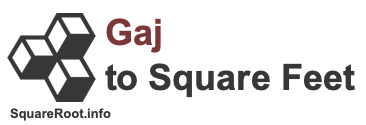100 gaj in square feetHere we will explain and show you how to convert 100 gaj to square feet. Before we continue, note that 100 gaj to square feet is the same as 100 gaj to sq ft, 100 gaj to ft2, and 100 gaj to ft².

Gaj and square feet are both measurements of an area, like a plot of land. One gaj is equal to 8.913592398096 square feet. Therefore, we can make the following gaj to square feet formula:

gaj × 8.913592398096
= square feet

Below is the math using our gaj to square feet formula to get 100 gaj in square feet.

= gaj × 8.913592398096
= 100 × 8.913592398096
= 891.3592398096
100 gaj ≈ 891.36 square feet

We have seen real estate brokers and others rounding up a gaj to nine square feet. Consequently, they simply multiply 100 gaj by nine to get it converted to square feet. In that case, this is how you would convert 100 gaj to square feet:

gaj × 9 = square feet
100 × 9 = 900
100 gaj = 900 square feet

Gaj to Square Feet Converter
Please enter another gaj area in the box below to have it converted to square feet.

Convert  gaj to square feet.

101 gaj in square feet
Here is the next area in gaj on our list that we have converted to square feet for you.